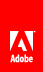# w

Determines the width of a given form design object.

#### Syntax

`Reference_Syntax.w( OBJECT param1 [, STRING param2 [, INTEGER param3 ] ] )`

#### Parameters

 param1 The fully qualified reference syntax expression of one of the following container XML Form Object Model objects: area , contentArea , draw , field , pageArea , subform . param2 (Optional) A string representing the unit type of the return value. If left blank, the default unit type is points. param3 (Optional) An integer representing a zero-based index value indicating the content area in which you want to obtain the object’s width. If left blank, the default value is 0 . This parameter allows you to calculate the width of an object that is distributed across multiple content areas, such as pages. For example, if you want to find the width of a subform object that spans multiple content areas, you would use this parameter to enumerate the width of the subform in each of the content areas and add the totals together.

#### Returns

The width of the form design object in the current content area.

Model

Object

XFA 2.1

#### JavaScript

```// Returns the width of a single instance of TextField1
xfa.layout.w(TextField1,"in");

// Calculates the width of Subform1 across two content areas and displays
// the total in a message box.
var iWidth = xfa.layout.w(Subform1,"in",0) + xfa.layout.w(Subform1,"in",1);
xfa.host.messageBox(iWidth);```

#### FormCalc

```// Returns the width of a single instance of TextField1
xfa.layout.w(TextField1,"in")

// Calculates the width of Subform1 across two content areas and displays
// the total in a message box.
var iWidth = xfa.layout.w(Subform1,"in",0) + xfa.layout.w(Subform1,"in",1)
xfa.host.messageBox(iWidth)```// Ethnio survey code removed